# Polynomials and Polynomial FunctionsPage 1

#### WATCH ALL SLIDES

Slide 1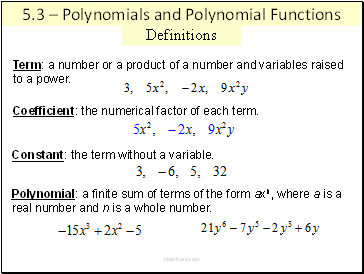## Definitions

5.3 – Polynomials and Polynomial Functions

Coefficient: the numerical factor of each term.

Constant: the term without a variable.

Term: a number or a product of a number and variables raised

to a power.

Polynomial: a finite sum of terms of the form axn, where a is a

real number and n is a whole number.

Slide 2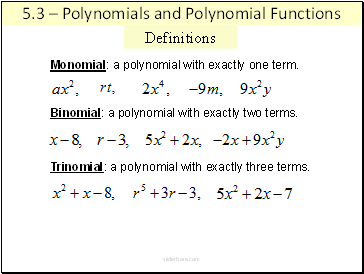Definitions

Monomial: a polynomial with exactly one term.

Binomial: a polynomial with exactly two terms.

Trinomial: a polynomial with exactly three terms.

5.3 – Polynomials and Polynomial Functions

Slide 3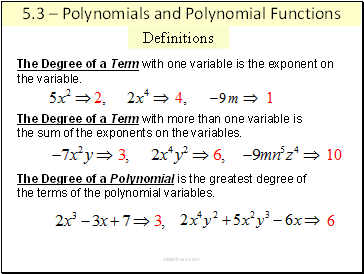Definitions

The Degree of a Term with one variable is the exponent on

the variable.

The Degree of a Term with more than one variable is the sum of the exponents on the variables.

The Degree of a Polynomial is the greatest degree of the terms of the polynomial variables.

5.3 – Polynomials and Polynomial Functions

Slide 4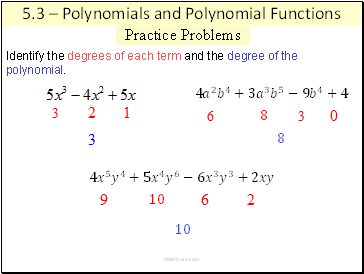## Practice Problems

Identify the degrees of each term and the degree of the polynomial.

5.3 – Polynomials and Polynomial Functions

4 𝑎 2 𝑏 4 +3 𝑎 3 𝑏 5 −9 𝑏 4 +4

8

8

4 𝑥 5 𝑦 4 +5 𝑥 4 𝑦 6 −6 𝑥 3 𝑦 3 +2𝑥𝑦

10

10

Slide 5Practice Problems

Evaluate each polynomial function

5.3 – Polynomials and Polynomial Functions

Slide 6## Multiplication

5.4 – Polynomials and Polynomial Functions

Multiplying Monomials by Monomials

Examples:

Slide 7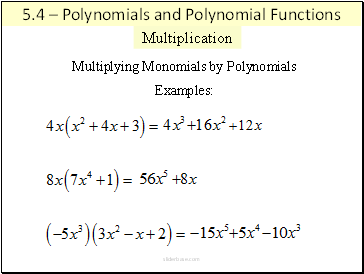Multiplying Monomials by Polynomials

Examples:

5.4 – Polynomials and Polynomial Functions

Multiplication

Slide 8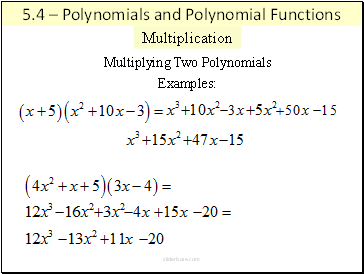Multiplying Two Polynomials

Examples:

5.4 – Polynomials and Polynomial Functions

Multiplication

Slide 9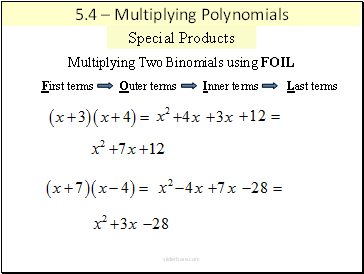## Special Products

5.4 – Multiplying Polynomials

Multiplying Two Binomials using FOIL

First terms

Last terms

Inner terms

Outer terms

Slide 10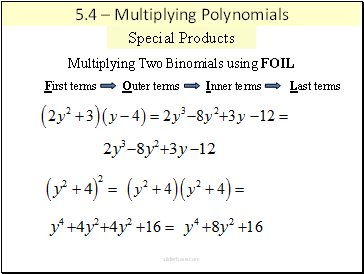Multiplying Two Binomials using FOIL

First terms

Last terms

Inner terms

Go to page:
1  2